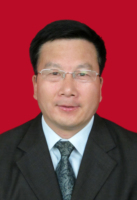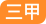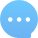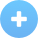首页 我的# 河南省中医院风湿疼痛科主任郭会卿教授发表的部分论文一览表（2）

郭会卿,李沛,杨豪,黄世金,赵灿,. 正骨手法CAI课件的开发和应用[J]. 中医药管理杂志,2008,(10).

李沛,郭现辉,郭会卿,曹玉净,吕秋霞,. 中医伤科影像教学课件的开发和应用[J]. 中国科教创新导刊,2008,(23).

李沛,郭会卿,冯仲锴,王上增,艾进伟,黄世金,. 中医骨伤人才培养模式的实践研究[J]. 中医药管理杂志,2008,(6).

郭会卿,李沛,杨豪,汪利合,曹玉净,黄世金,赵灿,. 《中医骨伤科学》精品课程建设规划及实施[J]. 中医药管理杂志,2008,(5).

杨豪,李沛,郭会卿,. 骨伤学科建设与研究生教育互动发展的探索[J]. 中医正骨,2007,(10).

郭会卿,李沛,王上增,. 强化学生技能训练,提高骨伤教学水平[J]. 中医正骨,2007,(7).

郭会卿,李沛,. 以评促建 加强内涵 提高骨伤学科教学质量[J]. 中医药管理杂志,2007,(6).

郭会卿,杨豪,李沛,曹玉净,. 中医骨伤临床型研究生实践技能培养改革探索[J]. 中医正骨,2007,(6).

郭会卿,. 针刺腰夹脊穴治疗腰椎间盘突出症疼痛43例[J]. 中医杂志,2007,(4).

史栋梁,杨豪,郭会卿,. 腰椎间盘突出症急性期非手术疗法进展[J]. 中医正骨,2007,(3).

李沛,郭会卿,王衍全,杨豪,. 中医骨伤学科建设的实践与体会[J]. 中医正骨,2007,(3).

郭会卿,李沛,. 影响骨伤专业学生临床实习效果原因分析及对策[J]. 中医药管理杂志,2007,(3).

郭会卿,. 附桂骨痛胶囊治疗膝关节骨性关节炎疗效观察[J]. 中成药,2007,(2).

郭会卿,. 劳损愈贴膜治疗腰椎间盘突出症的临床研究[J]. 中华中医药杂志,2007,(1).

郭会卿,. 中西医结合治疗髌骨软化症疗效观察[J]. 中医药学报,2006,(6).

郭会卿,. 针刺腰夹脊穴治疗腰椎间盘突出症的疗效观察[J]. 针灸临床杂志,2006,(11).

郭会卿,. 劳损愈贴膜的研制及临床应用观察[J]. 中医药临床杂志,2006,(5).

郭会卿,. 益肾蠲痹丸治疗顽痹150例疗效观察[J]. 时珍国医国药,2006,(10).

郭会卿,. 筋骨痛消丸合施沛特治疗膝骨性关节炎60例[J]. 陕西中医,2006,(9).

郭会卿,李沛,杨豪,. 中医骨伤专业后期教学模式的实践与探讨[J]. 中医正骨,2006,(8).

孟庆良,郭会卿,赵一静,. 益肾蠲痹汤合甲氨喋呤治疗类风湿性关节炎40例[J]. 中医研究,2006,(8).

郭会卿,. 中西医结合治疗膝关节退行性关节炎疗效观察[J]. 辽宁中医杂志,2006,(8).

郭会卿,. 娄多峰教授治疗类风湿性关节炎120例[J]. 中医研究,2006,(5).

吴振平,郭会卿,李沛,. 劳损愈贴膜外贴治疗腰椎间突出症临床观察[J]. 中医正骨,2005,(11).

郭会卿,吴振平,李沛,. 四联疗法治疗腰椎间盘突出症125例报告[J]. 中医正骨,2005,(11).

郭会卿. 推拨法配合劳损愈贴膜治疗髂腰韧带损伤54例临床观察[J]. 河北中医,2005,(6).

李沛,李莉,庄严,郭会卿,陈茜,安丙辰. 劳损愈贴膜的研制及安全性实验[J]. 中国中药杂志,2005,(9).

郭会卿. 点穴通经手法配合劳损愈贴膜外贴治疗腰肌劳损122例[J]. 河南中医,2005,(3).

李沛,郭会卿,申杰,丁松亭,杨豪,郭现辉,王云明,李现林,汪利合,韩冬冬. 劳损愈贴膜治疗腰肌劳损临床研究总结[J]. 中医正骨,2003,(5).

郭会卿 ,李沛 ,陈茜,郝铁来. 劳损愈贴膜抗炎镇痛活血作用的实验研究[J]. 中医正骨,2002,(5).

王学昌,郭会卿,李沛,程方荣. 水平面桌椅对颈肩腰背部的影响[J]. 中国骨伤,2002,(2).

郭会卿,吴振平,杨豪. 愈骨丹治疗股骨头缺血性坏死疗效观察[J]. 中医正骨,2001,(7).

王学昌,程方荣,郭会卿,刘百伟. 儿童尺桡骨远端骨折背侧移位的发病及其诊治[J]. 中医正骨,2001,(5).

李沛,郭会卿,丁松亭,杨豪,郭现辉,李现林,孙永强. 中医骨伤专业临床课教学改革的研究[J]. 中医正骨,2001,(5).

李沛,郭会卿,丁松亭,杨豪,李现林!(郑州450003),孙永强!(郑州450002). 中医骨伤专业临床教学改革实践[J]. 中医教育,2001,(3).

王学昌,郭会卿,李沛,程方荣. 水平面桌椅对脊柱的影响初探[J]. 河南中医药学刊,2001,(1).

李沛,韩冬冬,郭会卿. 腰突定痛贴配合推拿治疗腰椎间盘突出症105例[J]. 山东中医杂志,2001,(4).

李沛,郭会卿. 腰突定痛贴治疗腰椎间盘突出症的实验研究[J]. 山东中医杂志,2000,(11).

郭会卿,李沛,杨豪,杜玉兰,徐丹慧. 《中医伤科学》课程目标的建立及实施[J]. 中医正骨,1999,(7).

郭会卿. 颈痛贴治疗痹痛型颈椎病疗效观察[J]. 中医正骨,1999,(6).

杨豪,郭会卿,李沛,李根林,丁松亭,韩冬冬,史永振. 腰突定痛贴治疗腰椎间盘突出症疗效观察[J]. 中医正骨,1998,(3).

郭会卿. 肩周炎的历史沿革——兼谈保守治疗方法[J]. 河南中医,1998,(3).

孙锋,杨豪,罗小鹏,丁松亭,郭会卿. 平衡腰枕治疗腰肌劳损108例报告[J]. 河南中医,1998,(2).

杨豪,李沛,郭会卿. 中医药治疗腰椎间盘突出症近况[J]. 新中医,1997,(S1).

任小巧,蒋士卿,卢跃卿,罗小鹏,郭会卿,苗明三. 肝肾阴虚证与血脂、能量代谢、自由基反应关系探讨[J]. 河南中医,1996,(6).

郭会卿. 苏木合剂治疗急性软组织损伤的临床观察[J]. 河南中医,1996,(3).

李现林，郭会卿，康国喜. 苏木合剂治疗急性软组织损伤临床报告[J]. 河南中医药学刊,1996,(3).

赵本刚，孙科，王玉萍，郭会卿. 胃宁丸治疗消化性溃疡临床对比观察[J]. 河南中医,1995,(2).

郭会卿. 原发性骨质增生病因探讨[J]. 河南中医药学刊,1995,(6).

郭会卿，谢　松，李凯军. 创伤后关节僵硬临床治疗研究现状[J]. 中医正骨,1994,(3).问医生去挂号
App 内打开